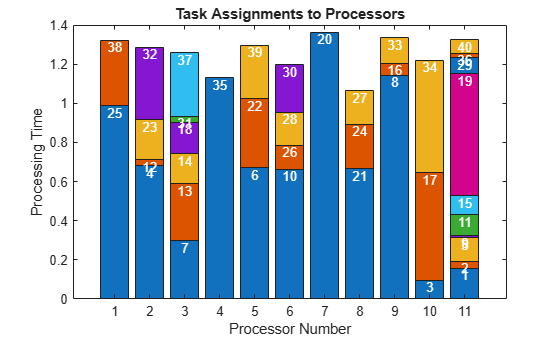# Minimize Makespan in Parallel Processing

This example involves a set of tasks to be processed in parallel. Each task has a known processing time. The makespan is the amount of time to process all of the tasks. This figure shows two processors; the height of each colored box represents the length of time to process a task. Each task can have a different run time on each processor.Your goal is to schedule tasks on processors so as to minimize the makespan.

### Problem Setup

This example has 11 processors and 40 tasks. The time for each processor to process each task is given in the array `processingTime`. For this example, generate random processing times.

```rng default % for reproducibility numberOfProcessors = 11; numberOfTasks = 40; processingTime = [10;7;2;5;3;4;7;6;4;3;1] .* rand(numberOfProcessors,numberOfTasks);```

`processingTime(i,j)` represents the amount of time that processor `i` takes to process task `j`.

To solve the problem using binary integer programming, create `process` as a binary optimization variable array, where `process(i,j) = 1` means processor `i` processes task `j`.

`process = optimvar('process',size(processingTime),'Type','integer','LowerBound',0,'UpperBound',1);`

Each task must be assigned to exactly one processor.

`assigneachtask = sum(process,1) == 1;`

To represent the objective, define a nonnegative optimization variable named `makespan`.

`makespan = optimvar('makespan','LowerBound',0);`

Compute the time that each processor requires to process its tasks.

`computetime = sum(process.*processingTime,2);`

Relate the compute times to the makespan. The makespan is greater than or equal to each compute time.

`makespanbound = makespan >= computetime;`

Create an optimization problem whose objective is to minimize the makespan, and include the problem constraints.

```scheduleproblem = optimproblem('Objective',makespan); scheduleproblem.Constraints.assigneachtask = assigneachtask; scheduleproblem.Constraints.makespanbound = makespanbound;```

### Solve Problem and View Solution

Solve the problem, suppressing the usual display.

```options = optimoptions(scheduleproblem,'Display',"off"); [sol,fval,exitflag] = solve(scheduleproblem,'Options',options)```
```sol = struct with fields: makespan: 1.3634 process: [11x40 double] ```
```fval = 1.3634 ```
```exitflag = OptimalSolution ```

The returned `exitflag` indicates that the solver found an optimal solution, meaning the returned solution has minimal makespan.

The returned makespan is 1.3634. Is this an efficient schedule? To find out, view the resulting schedule as a stacked bar chart. First, create a schedule matrix where row `i` represents the tasks done by processor `i`. Then, find the processing time for each entry in the schedule.

```processval = round(sol.process); maxlen = max(sum(processval,2)); % Required width of the matrix % Now calculate the schedule matrix optimalSchedule = zeros(numberOfProcessors,maxlen); ptime = optimalSchedule; for i = 1:numberOfProcessors schedi = find(processval(i,:)); optimalSchedule(i,1:length(schedi)) = schedi; ptime(i,1:length(schedi)) = processingTime(i,schedi); end optimalSchedule```
```optimalSchedule = 11×10 25 38 0 0 0 0 0 0 0 0 4 12 23 32 0 0 0 0 0 0 7 13 14 18 31 37 0 0 0 0 35 0 0 0 0 0 0 0 0 0 6 22 39 0 0 0 0 0 0 0 10 26 28 30 0 0 0 0 0 0 20 0 0 0 0 0 0 0 0 0 21 24 27 0 0 0 0 0 0 0 8 16 33 0 0 0 0 0 0 0 3 17 34 0 0 0 0 0 0 0 ⋮ ```

Display the schedule matrix as a stacked bar chart. Label the top of each bar with the task number.

```figure bar(ptime,'stacked') xlabel('Processor Number') ylabel('Processing Time') title('Task Assignments to Processors') for i=1:size(optimalSchedule,1) for j=1:size(optimalSchedule,2) if optimalSchedule(i,j) > 0 processText = num2str(optimalSchedule(i,j),"%d"); hText = text(i,sum(ptime(i,1:j),2),processText); set(hText,"VerticalAlignment","top","HorizontalAlignment","center","FontSize",10,"Color","w"); end end end```Find the minimum height of the stacked bars, which represents the earliest time a processor stops working. Then, find the processor corresponding to the maximum height.

`minlength = min(sum(ptime,2))`
```minlength = 1.0652 ```
`[~,processormaxlength] = max(sum(ptime,2))`
```processormaxlength = 7 ```

All processors are busy until time `minlength` = 1.0652. From the stacked bar chart, you can see that processor 8 stops working at that time. Processor `processormaxlength` = 7 is the last processor to stop working, which occurs at time `makespan` = 1.3634.